603 - 5885 1250 (4 lines) info@mes100.com
Select Page

Go to:

>cross section detailing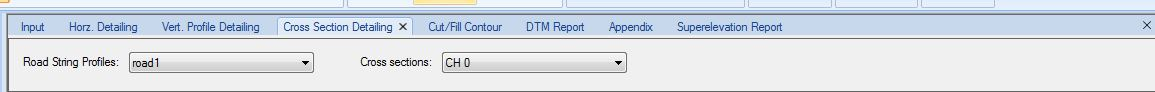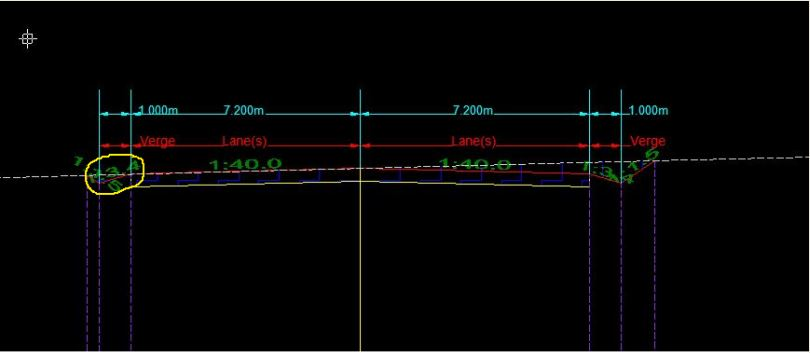As shown above, the verge slope is 1:3.4.

Go to:

Options >> Parameters >> Analysis >> Design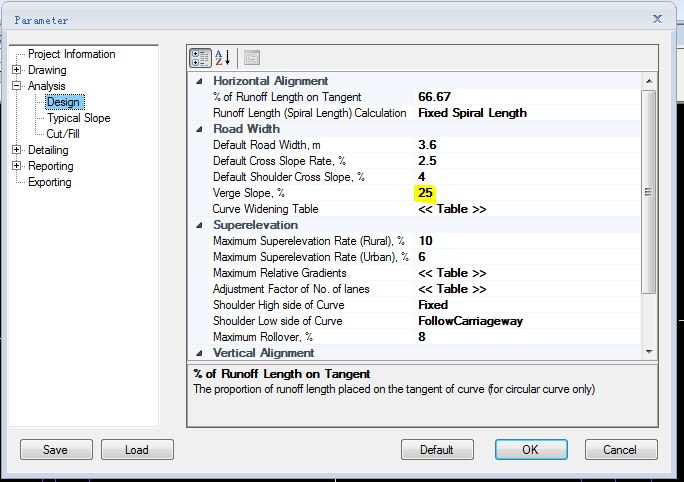As stated above, the verge slope is at 25% which supposedly give out the ratio in the cross section detailing at verge of 1:4.

Explanation:

1. The verge slope ratio at cross section is calculated cumulatively from the edge of the carriageway which includes the shoulder slope and verge slope as the edge of the carriageway is considered as reference point.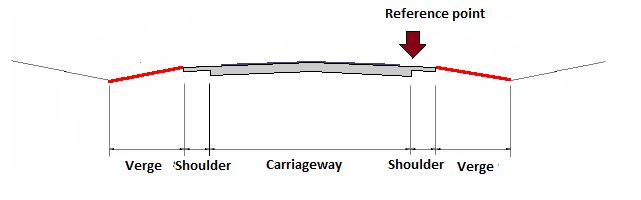2. In this case, shoulder cross slope and verge slope are set at 4% and 25%, respectively.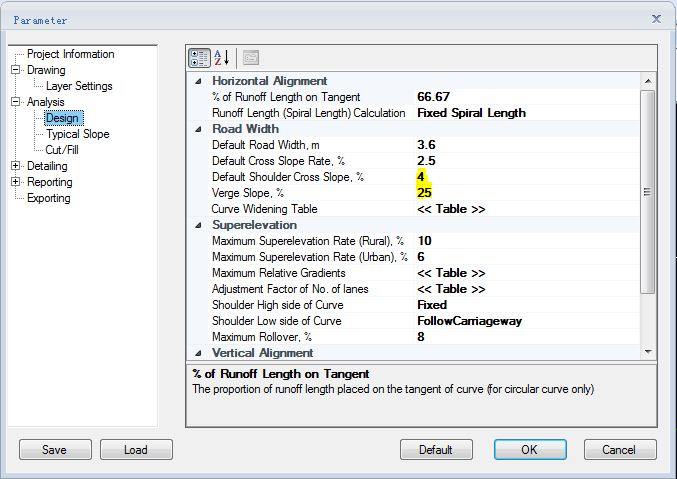1. Therefore, it is calculated by totalling the shoulder cross slope and verge slope (4+25) which gives out 29% equals to ratio of 1:3.4.